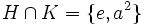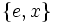Permutably complemented is not finite-intersection-closed

This article gives the statement, and possibly proof, of a subgroup property (i.e., permutably complemented subgroup) not satisfying a subgroup metaproperty (i.e., finite-intersection-closed subgroup property).
View all subgroup metaproperty dissatisfactions | View all subgroup metaproperty satisfactions|Get help on looking up metaproperty (dis)satisfactions for subgroup properties
Get more facts about permutably complemented subgroup|Get more facts about finite-intersection-closed subgroup property|

Statement

It is possible to have a group$G$ and two permutably complemented subgroups$H, K \le G$ such that$H \cap K$ is not permutably complemented in$G$.

Proof

Example of the dihedral group

Further information: dihedral group:D8

Suppose$G$ is the dihedral group of order eight:$G = \langle a,x \mid a^4 = x^2 = e, xax^{-1} = a^{-1} \rangle$.

Consider the two subgroups:$H = \langle a^2, x \rangle = \{ e, a^2, x, a^2x \}, \qquad K = \langle a^2, ax \rangle = \{ e, a^2, ax, a^3x \}$.

Then:$H \cap K = \{ e, a^2 \}$.

We have:

•$H$ is a permutably complemented subgroup of$G$: The subgroup$\{ e, ax \}$ is a permutable complement to$H$ in$G$.
•$K$ is a permutably complemented subgroup of$G$: The subgroup$\{ e, x\}$ is a permutable complement to$K$ in$G$.
•$H \cap K$ is not permutably complemented in$G$: This can be seen by direct inspection, but also follows from the more general fact that in a nilpotent group any nontrivial normal subgroup intersects the center nontrivially. Here,$H \cap K$ is the center, and if it has a permutable complement, that subgroup must be a nontrivial normal subgroup, leading to a contradiction.